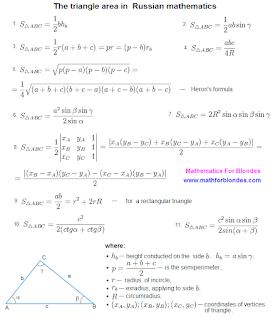## 1/29/2011

### The triangle area

The triangle area concerns that number of school problems which very often should be solved and in the further life. Now we speak not about the area of a triangle Bermudas which at desire can be calculated (by the way, it makes more than one million square kilometres), and not about the area of a triangle love which basically it is impossible to calculate. We speak about the area of that triangle which is a geometrical figure. By the way, from a figure of the blonde of mathematics something took for the favourite triangles. But about it another time.

Today we will look one eye at formulas of a triangle for an area finding. The triangle area in Wikipedia is given in the form of the whole heap of formulas. I стырил them therefrom also have made in the most unscrupulous image for you a crib on geometry with formulas of the area of a triangle and a portrait of the hero of the festivities - a triangle. This crib on favourite our mathematics can be downloaded free of charge - the right button of a mouse (fiiii!) "to keep drawing as". This crib remains at you in the computer.The triangle area

Let's begin with a triangle picture. In different textbooks there can be different designations of tops, the parties and triangle corners. Therefore, before it is stupid to apply formulas from a crib to the decision of the problems, compare all designations of the parties, corners and tops of triangles. Quite probably that some letters in formulas to you should be changed, what on a crib and in your textbook there were identical designations.

Now about formulas. Under figure 1 (one) costs the most widespread formula for a finding of the area of a triangle in length of the party of a triangle and the height of a triangle lowered on this party. The area of a triangle to half of product of the party of a triangle on height equals.

The second formula allows to find the triangle area on length of two parties and a corner between them. At the formula there is a sine of the angle the scale for which value it is necessary to search under the trigonometrical table or to calculate on the calculator.

The third formula allows to find the triangle area on three parties and radius of the entered circle. Equal-signs in this formula divide different variants of this formula in which the triangle semiperimeter, radius of the extraentered circle, concerning is applied by one of the parties. With these features we somehow will make an effort understand. For now below our crib it is possible to find that designation who is who in our triangular zoo. And formulas for calculation of height and triangle semiperimeter.

On lengths of three parties and radius of the described circle it is possible to find the area by means of the fourth formula.

My most favourite formula - Geron's formula - at number five. This formula allows to find the triangle area on three parties. Than so this Heron's formula is good? On work I with its help calculated the area of almost any geometrical figure. For example, if the room had the form of a wrong polygon was to measure lengths of walls and distance between room corners (lengths of the parties and distance between polygon tops enough). Then under the formula of Gerona the area of triangles into which it is possible to break any polygon was calculated. The sum of the areas of triangles gave the polygon area, that is the room area. In a crib the Heron's formula is presented in two variants - a finding of the area of a triangle through semiperimeter and in lengths of the parties.

Further we can find the triangle area on one party and three corners (the formula 6), on radius of the described circle and three corners (the formula 7), on co-ordinates of tops of a triangle (the formula 8). In last formula vertical sticks in numerator designate the number module, after all the area cannot be negative are mathematicians know even.

The area of a rectangular triangle (the formula 9) can be found as half of product of legs of a triangle or through radiuses of the entered and described circle.

In conclusion of our excursion on Placco De the Triangle we can find the triangle area on the party and two corners, using cotangent (the formula 10) or sine (the formula 11) these corners.